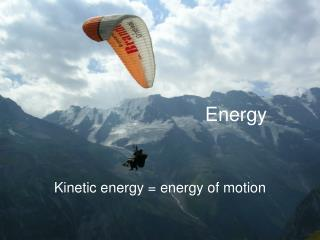# Energy - PowerPoint PPT PresentationDownload PresentationEnergy

EnergyDownload Presentation## Energy

- - - - - - - - - - - - - - - - - - - - - - - - - - - E N D - - - - - - - - - - - - - - - - - - - - - - - - - - -
##### Presentation Transcript

1. Energy Kinetic energy = energy of motion

2. Energy Potential energy = stored energy 1. energy of position due to gravity

3. Energy Potential energy = stored energy 2. energy stored in chemical bonds

4. Law of Conservation of Energy Energy can not be created or destroyed, only converted from one form to another.

5. When energy is RELEASED in a chemical reaction, it is called…. EXOTHERMIC

6. When energy is ABSORBED in a chemical reaction, it is called…. ENDOTHERMIC

7. Units of Energy Energy is measured in JOULES Also in calories or kilocalories (food) How many calories are in a kilocalorie?

8. Units of Energy How many calories in the hamburger? 420 kilocalories

9. What is temperature? A measure of the average kinetic energy of the particles in a sample of matter. All atoms are moving! High temperature = moving faster

10. Once again…… TEMPERATURE = AVERAGE KINETIC ENERGY

11. Temperature Scales Celcius: water freezes water boilsZero degrees 100 degrees

12. Temperature Scales Kelvin: 0 K = absolute zero (-460 oF) all motion of particles stops! Water freezes @ 273 K Water boils @ 373 K Kelvin never goes below zero (no negative numbers!)

13. Temperature Scales Kelvin = Celsius + 273 Celsius = Kelvin – 273 If it is 32O Celsius, what temperature is it in Kelvin? If it is 100 Kelvin, what temperature is it in Celsius?

14. Temperature Scales

15. Standard Temperature 0 degrees Celsius 273 Kelvin (in Table A)

16. At what temperature does liquid nitrogen boil? • 77 Kelvin • -195 Celsius • -320 Farenheit

17. What is Heat? Heat is the sum total of all the kinetic energy in a sample of matter. How is heat different from temperature???

18. Heat ≠ Temperature Both the water in the bath tub and in the cup are at 25 oC Which contains more HEAT?

19. Heat Capacity The amount of energy (in JOULES) needed to raise the temperature of the sample by 1 oC Which water sample has a higher heat capacity?

20. Specific Heat Capacity The amount of energy (in JOULES) needed to raise the temperature of 1 gram of the sample by 1 oC Which water sample has a higher specific heat ?

21. Specific Heat Specific heat measures how easily something can absorb (or then lose) heat. LOW specific heat = little energy for something to heat up. HIGH specific heat = lots of energy to heat something up Every substance has its own unique specific heat value.

22. Specific Heat Capacity In your experience, which substance below has a higher specific heat capacity (takes more energy to heat it up?)